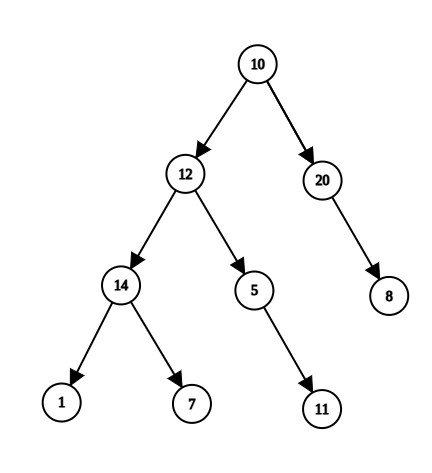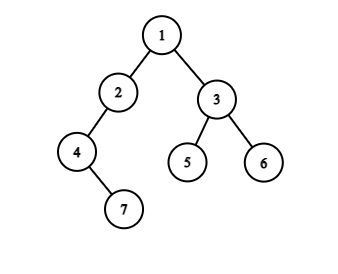New update is available. Click here to update.

# Ancestors in Binary Tree

Posted: 2 Dec, 2020
Difficulty: Moderate

## PROBLEM STATEMENT

#### A binary tree is a hierarchical data structure in which each node has at most two children.

##### Example:``````Here, for ‘k’ = 7, the output will be 14 12 10.
``````
##### Input Format:
``````The first line contains an Integer 'T' which denotes the number of test cases or queries to be run. Then the test cases follow.

The first line of each test case contains the elements of the tree in the level order form separated by a single space.
If any node does not have a left or right child, take -1 in its place. Refer to the example below.

The second line of each test case contains a single integer ‘k’, denoting the value of the node whose ancestors you have to found.

The given node may not be present in the tree. But it is guaranteed that there will be a maximum of one node with the value ‘k’.
``````
##### Example:
``````Elements are in the level order form. The input consists of values of nodes separated by a single space in a single line. In case a node is null, we take -1 in its place.

For example, the input for the tree depicted in the below image would be :
````````````1
2 3
4 -1 5 6
-1 7 -1 -1 -1 -1
-1 -1

Explanation :
Level 1 :
The root node of the tree is 1

Level 2 :
Left child of 1 = 2
Right child of 1 = 3

Level 3 :
Left child of 2 = 4
Right child of 2 = null (-1)
Left child of 3 = 5
Right child of 3 = 6

Level 4 :
Left child of 4 = null (-1)
Right child of 4 = 7
Left child of 5 = null (-1)
Right child of 5 = null (-1)
Left child of 6 = null (-1)
Right child of 6 = null (-1)

Level 5 :
Left child of 7 = null (-1)
Right child of 7 = null (-1)

The first not-null node (of the previous level) is treated as the parent of the first two nodes of the current level. The second not-null node (of the previous level) is treated as the parent node for the next two nodes of the current level and so on.

The input ends when all nodes at the last level are null (-1).

Note: The above format was just to provide clarity on how the input is formed for a given tree.

The sequence will be put together in a single line separated by a single space. Hence, for the above-depicted tree, the input will be given as:

1 2 3 4 -1 5 6 -1 7 -1 -1 -1 -1 -1 -1
``````
##### Output Format:
``````For each test case, print a list of integers denoting the ancestors of the given node in reverse order(lowest ancestor first). If the node is not present in the tree, then return -1.

Output for each test case will be printed in a separate line.
``````
##### Note:
``````You are not required to print anything, it has already been taken care of. Just implement the function.
``````
##### Constraints:
``````1 <= T <= 100
1 <= N <= 5 * 10^3
0 <= X <= 10^9

Where ‘X’ is the value at the node.

Time Limit: 1 sec.
``````
SIMILAR PROBLEMS

Ninja and Tree

Posted: 5 Feb, 2022
Difficulty: Ninja

Kth Largest Element in BST

Posted: 12 Mar, 2022
Difficulty: Moderate

Height of Binary Tree

Posted: 22 Apr, 2022
Difficulty: Easy

Min Heap

Posted: 5 May, 2022
Difficulty: Moderate

Locked Binary Tree

Posted: 12 May, 2022
Difficulty: Easy